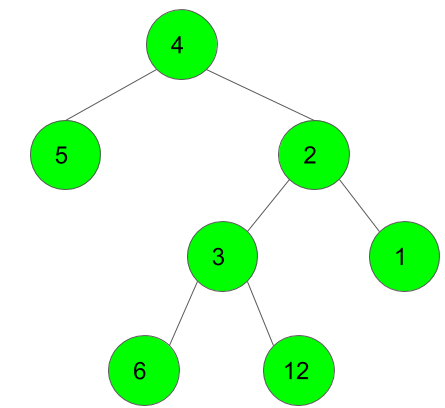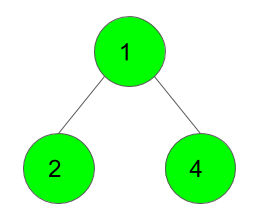XDAYS

:

HOUR

:

MINS

:

SEC

Copied to Clipboard
Maximum GCD of siblings of a binary tree
Easy Accuracy: 51.16% Submissions: 1559 Points: 2

Given a 2d list that represents the nodes of a Binary tree with N nodes, the task is to find the maximum GCD of the siblings of this tree without actually constructing it.
Note: If there are no pairs of siblings in the given tree, print 0. Also, if given that there's an edge between a and b in the form of {a,b} in the list, then a is the parent node.

Example 1:

Input:
N = 7
arr = {{4, 5}, {4, 2}, {2, 3}, {2, 1}, {3, 6}, {3, 12}}
Output:
6
Explanation:For the above tree, the maximum GCD
for the sibilings is 6, formed for the
nodes 6 and 12 for the children of node 3.

Example 2:
Input:
N = 3
arr[] = {{1,2}, {1,4}}
Output :
2
Explanation:For the above tree, the maximum GCD
for the sibilings is 2, formed for the
nodes 2 and 4 for the children of node 1.


You don't need to read input or print anything. Your task is to complete the function maxBinTreeGCD() which takes an integer N and a 2-d list denoting the edges as input and returns the maximum GCD of sibilings of the tree.

Expected Time Complexity: O(E*logE), where E is the number of edges in the Tree.
Expected Auxiliary Space: O(1)

Constraints:
1 ≤ N ≤ 105
There might be edges with similar values

We are replacing the old Disqus forum with the new Discussions section given below.

### Editorial

We strongly recommend solving this problem on your own before viewing its editorial. Do you still want to view the editorial?

#### My Submissions: